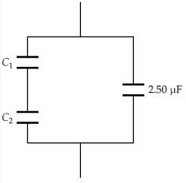# Find the total capacitance of the combination of capacitors shown in the figure below

Find the total capacitance of the combination of capacitors shown in the figure below. (C1 =0.295 μF, C2 =24.0 μF) Answer in μF